Math Concepts

# Fraction and Decimals

498 views

## Table of Contents

 1 Introduction to Fractions 2 Types of Fractions 3 Finding the lowest form of fractions 4 Equivalent fractions 5 Arithmetic Operations on Fractions 6 Fractions in our Daily Life 7 Decimals 8 Problems 9 FAQs

30th September 2020

Reading Time: 5 Minutes

## Introduction To Fraction

The word ‘Fraction’ came from the  Latin word ‘ Fractus’ which means ‘a breaking’ or’ a fragment’. Example a part of a whole Pizza.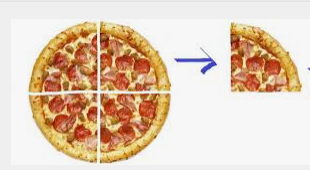### Definition of Fraction

A Fraction is described as ‘ how many parts of a certain whole there are?’ for example- one-third, two-fourth.

### Notation of Fraction

The notation of a Fraction in Mathematics is nothing but a number that can be written in the form of ‘a/b’.  The number which is written at the top (a), is called the numerator, and the number at the bottom of the bar called the  Denominator.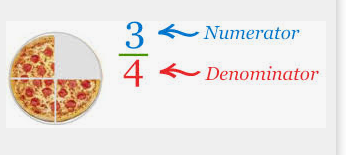When we are writing a Fraction,e.g. 3/5 or three fifth or the denominator represents the total number of parts into which a whole divided. And the number above the bar is called the numerator, which is the number of parts of the unit which has been taken.  For example, Sam has a chocolate bar that has 5 pieces and Sam ate 3 pieces from it. So the fractional form of the amount of Chocolate ate by Sam will be ⅗ or three fifths.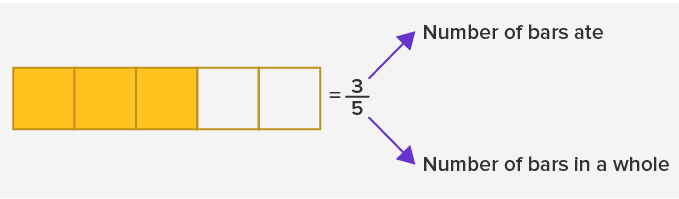The Notation of Fraction that we write today is of Indian origin and later it has been taken by the Egyptian mathematicians.

Also read:

How to add Fractions with different combinations?

## Types of fraction

There are different kinds of Fractions, they are as follows:

1. Like fraction: The fractions that have the same Denominator, are called Like fraction.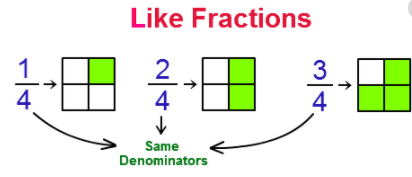2.Same Numerators Fraction: When different Fractions have the same Numerators but different Denominators, is called the same Numerator fraction.3. Unit Fraction: When the numerator of a fraction is one (1), is called a Unit fraction.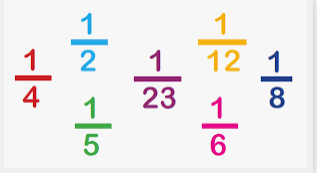4.Proper Fraction: A fraction in which the denominator is greater than the numerator, is called Proper fraction. A proper fraction is a part of a whole and always less than 1.
⅜ <1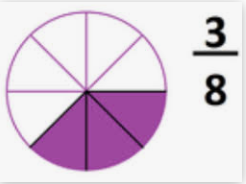5. Improper Fraction: A fraction in which the numerator is greater than the denominator, is called an Improper Fraction. It is greater than a whole or 1.

5/4>1.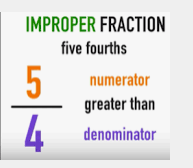6. Mixed Fraction: When the improper fraction is written as the combination of a whole number as well as a proper fraction, is called a mixed fraction. A mixed fraction is nothing but the different representation of Improper fraction. It is also greater than a whole or 1.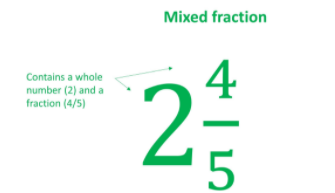Mixed fraction to Improper fraction:
For instance,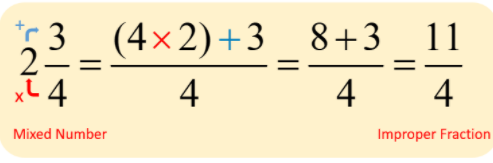Improper fraction to mixed fraction: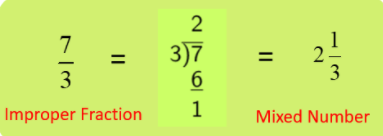## Finding the lowest form of Fraction:

The lowest form of a fraction is the fraction where the numerator and the denominator do not have any common divisor.

In other words, the lowest form of a fraction is nothing but a fraction where the numerator and denominator are a coprime number.  To reduce the fraction to its lowest form we need to divide the numerator and denominator by their  GCF.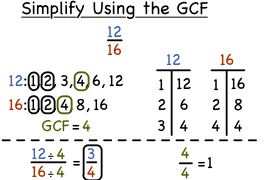## Equivalent Fraction:

Equivalent Fraction means the Fractions which are of the same value but looks different.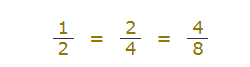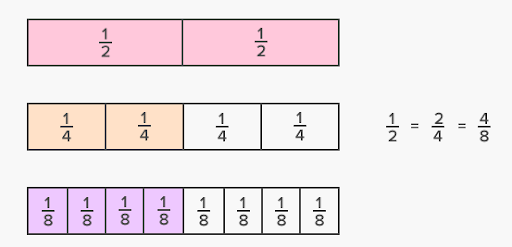Equivalent fractions are the same because if we multiply or divide the numerator and denominator by the same number, the fraction remains the same.

If we multiply by the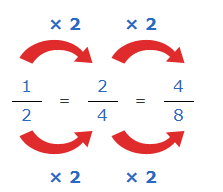If we divide by the same number,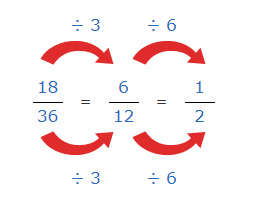## Arithmetic operations involving Fraction:

• Addition  and Subtraction: When the denominators are the same,

If the denominators are the same, only the numerator needed to be added or subtracted and the denominator will remain the same.

• Addition and Subtraction: When the denominators are different,

When the fractions have unlike denominators or different denominators, first we have to convert the denominators as a common denominator,

2/3  + 3/7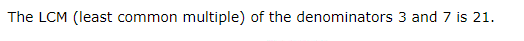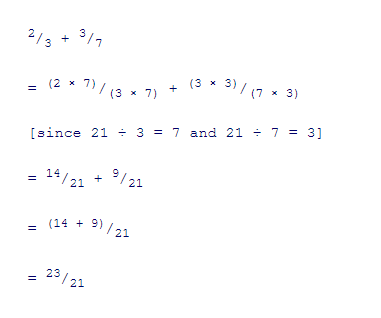2/5 - 1/10
The L.C.M. of the denominators 10 and 5 =10.
2/5 = (2 × 2)/(5 × 2) = 4/10
1/10 = (1 × 1)/(10 × 1) = 1/10
So, 2/5 - 1/10
= 4/10 - 1/10
= (4 - 1)/10
= 3/10

### Multiplication of two or more fractions:

In the multiplication of two or more like or unlike fractions, the Numerator multiplied by the other numerator and denominator will be multiplied by another denominator.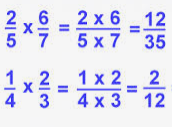### Division of two or more Fractions:

The division of one fraction by another is can be done by inverting the divisor and then multiply.

1/8 ÷ 1/4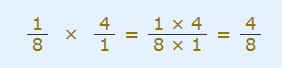## Fractions in our Daily life:

Some examples of Fraction in our daily life are:
1. A Pizza is an example of a fraction where each of the pieces is a part of a whole.2. The fruit is another example of a fraction. Every time we cut any fruit into a piece, we are making a part of a whole.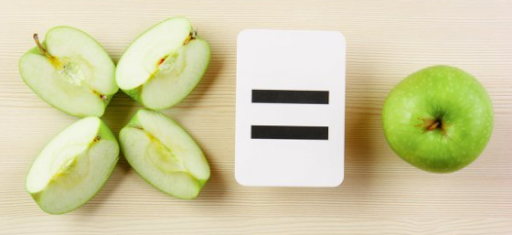## Decimals

The decimal number system is also known as the ‘Base-ten’ positional numeral system and also called Denary. In simpler meaning, a decimal fraction is a fraction where the denominator is either ten or the higher power of ten such as 10, 100,1000, etc.
The decimal fraction is represented as  a/10n

Where n=Natural Number.

### Decimal notation:

The decimal or decimal number identified by a separator called ’Decimal point’ or  “.”. Such as 3.14 is the approximate decimal value of pi \begin{align}\pi \end{align} =3.14

### History of decimals:

The decimal fraction was developed by the Chinese in the 4th century BCE.

### Types of Decimals:

There are 2 types of Decimals,
1. Non-recurring decimals or terminal decimals: Non-recurring decimals mean it does not reoccur.  E.g 0.5, 0.25 etc.
2. Recurring decimals or Non-terminal decimals: It means that it reoccurs. E.g.0.222…, 0.3333…., 0.232323….

The relation between Fraction and decimal:

For instance, a decimal number  12.4547, where 12is a whole number and 4547 is the decimal part or fractional part.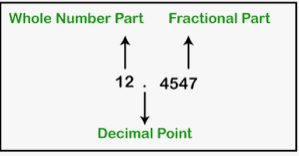• Decimals fraction based on the power of 10. So when we move left to right we have to divide the place value of the digits by 10. The place value after the decimals is Tenth, Hundredth, Thousandth, and so on.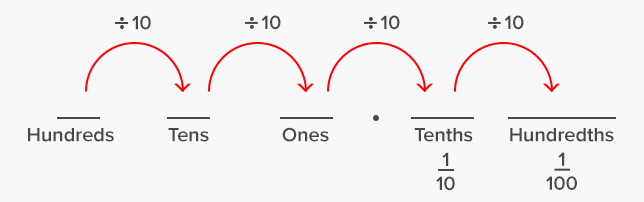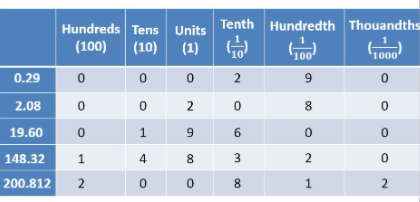• Decimal numbers of From Mixed Fraction.The expanded form of decimal numbers: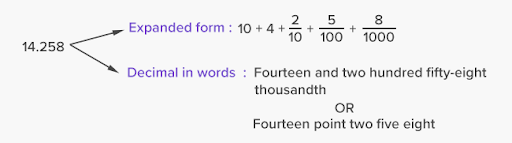Conversion of Decimals number from fraction:

To find the decimal number from its fraction we can use the following method-

• Long Division method: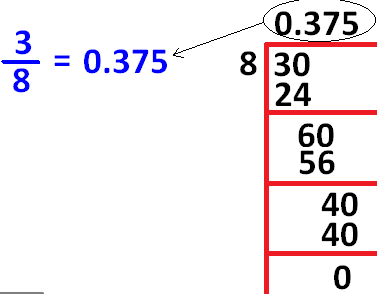• When we will divide a number by another number where the remainder is coming, we will add a decimal point in the quotient line. And for taking decimal point we can add one zero in each step.

Other methods:

1. We have to find a number that can be multiplied to the numerator and denominator to get 10,100, 1000 ...

¾ to decimal number
We have to multiply 4 by 25 to get 100  as Denominator.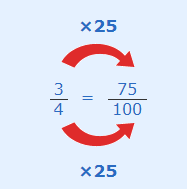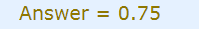2.  Then we have to write down the number after putting the decimal point, one space from the right side for every zero in the denominator.

## Problems on Fraction and decimals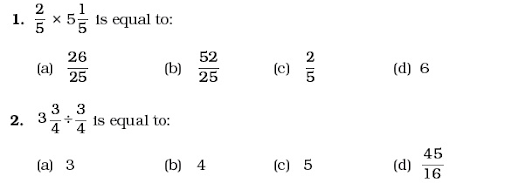3.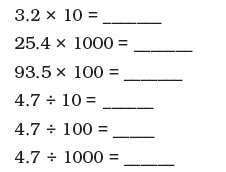4.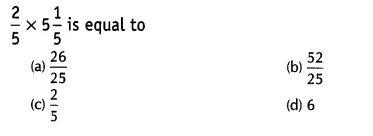5.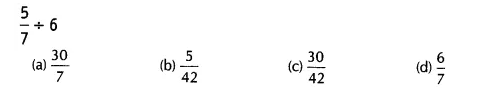## What is the difference between a decimal & a whole number?

Whole numbers specify how many elements there are in a collection of objects while decimals are a part of the whole numbers.

## How to add and subtract fractions?

For like fractions, add/subtract the numerators and retain the same denominator. For adding unlike fractions, take the LCM of the denominators and convert the addends to equivalent fractions and add them as like fractions. Reduce to its lowest term if needed.
What decimal of an hour is a second?
=1/(60×60)=1/3600=0.00027

## How do you multiply fractions?

• To multiply any two fractions, we can follow the steps below.
• Multiply the numerators
• Multiply the denominators
• Simplify to the lowest form

## How to divide fractions?

A simple way to explain how to divide fractions is :

1. Flip the reciprocal of the divisor.
2. Change the division sign into a multiplication sign
3. Multiply it with the dividend.

Related Articles
GIVE YOUR CHILD THE CUEMATH EDGE
Access Personalised Math learning through interactive worksheets, gamified concepts and grade-wise courses
Learn More About Cuemath